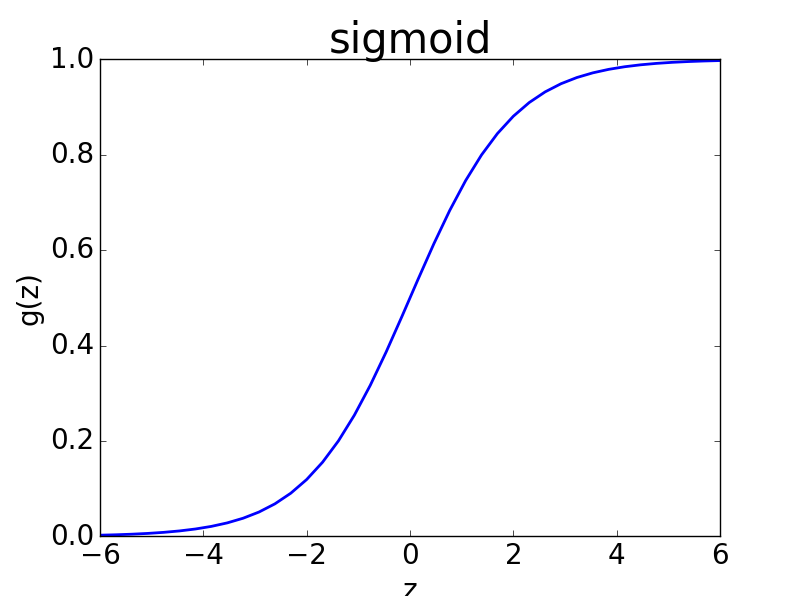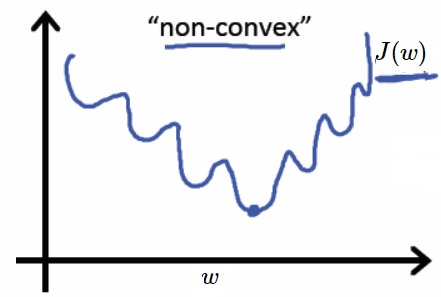什么是逻辑回归

logistic回归又称logistic回归分析，是一种广义的线性回归分析模型

如何处理因变量取值离散的情况

$\frac{y}{1-y}（式1.0）$

$\ln(\frac{y}{1-y})（式1.1）$

$\ln(\frac{y}{1-y})=w^Tx+b（式1.2）$

$y=\frac{1}{1+e^{-(w^Tx+b)}}(式1.3)$如何求解w、b$\ln(\frac{p(y=1|x)}{p(y=0|x)})=w^Tx+b（式2.0)$

$p(y=1|x)+p(y=0|x)=1（式2.1)$

$p(y=1|x)=\frac{e^{w^Tx+b}}{1+e^{w^Tx+b}}（式2.2） \\ p(y=0|x)=\frac{1}{1+e^{w^Tx+b}} （式2.3）$

• 1、m个样本数据之间互相独立
• 2、已知样本的概率密度函数（式2.2，式2.3）

$\iota(w,b)=\sum_{i=1}^m\ln(y_i|x_i;w,b)（式2.4）$

$\ln(y_i|x_i;w,b)=y_ip(y_i=1|x_i)+(1-y_i)p(y_i=0|x_i)（式2.5）$

$\iota(w,b)=\sum_{i=1}^m(\ln(y_ie^{w^Tx_i+b}+1-y_i)-\ln(1+e^{w^Tx_i+ b})) (式2.6)$

$\iota(w,b)=\sum_{i=1}^m(y_i(w^Tx_i+b)-\ln(1+e^{w^Tx_i+ b})) (式2.7)$

$\iota(w,b)=\sum_{i=1}^m(-y_i(w^Tx_i+b)+\ln(1+e^{w^Tx_i+ b})) (式2.8)$

梯度下降法的推导

$x_i$表示$(x_{i1},x_{i2},....,x_{in})$，即数据具有n个特征，$w$表示$(w_1,w_2,.....,w_n)$，共有m组数据，$y_i$表示第i组数据的取值，式2.8对$w、b$求导得
\begin{aligned} & \frac{\partial \iota}{\partial w_1}=\sum_{i=1}^m(-y_{i}x_{i1})+\sum_{i=1}^m(\ln(1+e^{w^Tx_i+b})x_{i1})\\ & \frac{\partial \iota}{\partial w_2}=\sum_{i=1}^m(-y_{i}x_{i2})+\sum_{i=1}^m(\ln(1+e^{w^Tx_i+b})x_{i2})\\ &.....\\ &\frac{\partial \iota}{\partial w_n}=\sum_{i=1}^m(-y_{i}x_{in})+\sum_{i=1}^m(\ln(1+e^{w^Tx_i+b})x_{in})\\ &\frac{\partial \iota}{\partial b}=\sum_{i=1}^m(-y_{i})+\sum_{i=1}^m(\ln(1+e^{w^Tx_i+b})) \end{aligned}

$\left\{ \begin{matrix} \frac{\partial \iota}{\partial w_1}\\ \frac{\partial \iota}{\partial w_2}\\ .\\ .\\ \frac{\partial \iota}{\partial w_n}\\ \frac{\partial \iota}{\partial b} \end{matrix} \right\} = \left\{ \begin{matrix} x_{11} & x_{21} & ... & x_{m1} \\ x_{12} & x_{22} & ... & x_{m2} \\ . & . & . &.\\ . & . & . &.\\ x_{1n} & x_{2n} & ... & x_{mn} \\ 1 & 1 & 1 &1\\ \end{matrix} \right\} \left\{ \begin{matrix} -y_1\\ -y_2\\ .\\ .\\ -y_m \end{matrix} \right\}+ \left\{ \begin{matrix} x_{11} & x_{21} & ... & x_{m1} \\ x_{12} & x_{22} & ... & x_{m2} \\ . & . & . &.\\ . & . & . &.\\ x_{1n} & x_{2n} & ... & x_{mn} \\ 1 & 1 & 1 &1\\ \end{matrix} \right\} \left\{ \begin{matrix} \ln(1+e^{w^Tx_1+b})e^{w^Tx_1+b} \\ \ln(1+e^{w^Tx_2+b})e^{w^Tx_2+b} \\ . \\ . \\ \ln(1+e^{w^Tx_m+b})e^{w^Tx_m+b} \\ \end{matrix} \right\}$

$X^T=\left\{ \begin{matrix} x_{11} & x_{21} & ... & x_{m1} \\ x_{12} & x_{22} & ... & x_{m2} \\ . & . & . &.\\ . & . & . &.\\ x_{1n} & x_{2n} & ... & x_{mn} \\ 1 & 1 & 1 &1\\ \end{matrix} \right\}$
$Y=\left\{ \begin{matrix} y_1\\ y_2\\ .\\ .\\ y_m \end{matrix} \right\}$
$H= \left\{ \begin{matrix} \ln(1+e^{w^Tx_1+b})e^{w^Tx_1+b} \\ \ln(1+e^{w^Tx_2+b})e^{w^Tx_2+b} \\ . \\ . \\ \ln(1+e^{w^Tx_m+b})e^{w^Tx_m+b} \\ \end{matrix} \right\}$
$w$的更新值$w^,$可表示为：

$w^,=w-\alpha( X^T(-Y)+X^TH)$